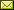## The Almost Equivalence by Asymptotic Probabilities for Regular Languages and Its Computational Complexities

 Yoshiki Nakamura (Tokyo Institute of Technology)

 We introduce p-equivalence by asymptotic probabilities, which is a weak almost-equivalence based on zero-one laws in finite model theory. In this paper, we consider the computational complexities of p-equivalence problems for regular languages and provide the following details. First, we give an robustness of p-equivalence and a logical characterization for p-equivalence. The characterization is useful to generate some algorithms for p-equivalence problems by coupling with standard results from descriptive complexity. Second, we give the computational complexities for the p-equivalence problems by the logical characterization. The computational complexities are the same as for the (fully) equivalence problems. Finally, we apply the proofs for p-equivalence to some generalized equivalences.

In Domenico Cantone and Giorgio Delzanno: Proceedings of the Seventh International Symposium on Games, Automata, Logics and Formal Verification (GandALF 2016), Catania, Italy, 14-16 September 2016, Electronic Proceedings in Theoretical Computer Science 226, pp. 272–286.
Published: 13th September 2016.

 ArXived at: http://dx.doi.org/10.4204/EPTCS.226.19 bibtex PDF
References in reconstructed bibtex, XML and HTML format (approximated).
 Comments and questions to:eptcs@eptcs.org For website issues:webmaster@eptcs.org